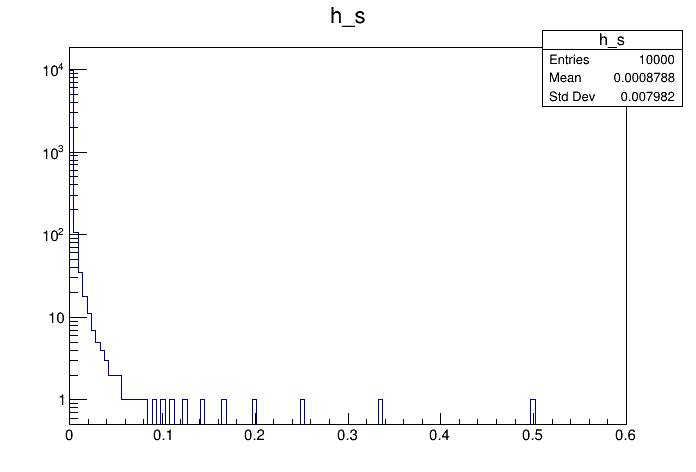# Df 0 1 1_ R O O T Data Source¶

This tutorial illustrates how use the RDataFrame in combination with a RDataSource. In this case we use a TRootDS. This data source allows to read a ROOT dataset from a RDataFrame in a different way, not based on the regular RDataFrame code. This allows to perform all sorts of consistency checks and illustrate the usage of the RDataSource in a didactic context.

Author: Danilo Piparo
This notebook tutorial was automatically generated with ROOTBOOK-izer from the macro found in the ROOT repository on Saturday, January 18, 2020 at 01:33 AM.

In :
import ROOT

Welcome to JupyROOT 6.19/01


A simple helper function to fill a test tree: this makes the example stand-alone.

In :
def fill_tree(treeName, fileName):
tdf = ROOT.ROOT.RDataFrame(10000)
tdf.Define("b1", "(int) tdfentry_").Snapshot(treeName, fileName)


We prepare an input tree to run on

In :
fileName = "df011_rootDataSource_py.root"
treeName = "myTree"
fill_tree(treeName, fileName)


Create the data frame

In :
MakeRootDataFrame = ROOT.ROOT.RDF.MakeRootDataFrame

d = MakeRootDataFrame(treeName, fileName)


Now we have a regular RDataFrame: the ingestion of data is delegated to the RDataSource. At this point everything works as before.

In :
h = d.Define("x", "1./(b1 + 1.)").Histo1D(("h_s", "h_s", 128, 0, .6), "x")


Now we redo the same with a RDF and we draw the two histograms

In :
c = ROOT.TCanvas()
c.SetLogy()
h.DrawClone()

Out:
<ROOT.TH1D object ("h_s") at 0x557d2a75fcd0>

Draw all canvases

In :
from ROOT import gROOT
gROOT.GetListOfCanvases().Draw()